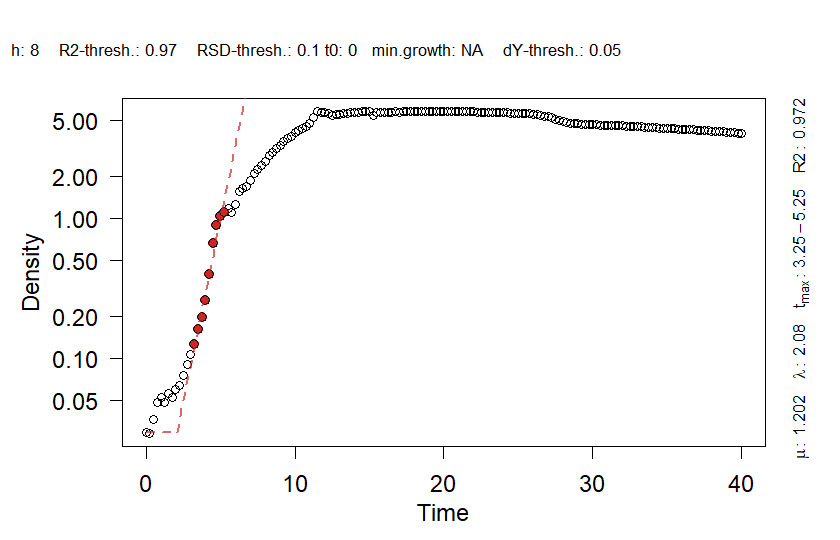Please note: This vignette will be updated from time to time when new features are implemented. Please find the most recent version at the QurvE GitHub repository.

# Introduction

In virtually all disciplines of biology dealing with living organisms, from classical microbiology to applied biotechnology, it is routine to characterize the growth of the species under study. QurvE provides a suite of analysis tools to make such growth profiling quick, efficient, and reproducible. In addition, it allows the characterization of fluorescence data for, e.g., biosensor characterization in plate reader experiments (further discussed in the vignette Quantitiative Fluorescence Curve Evaluation with Package QurvE). All computational steps to obtain an in-depth characterization are combined into user-friendly workflow functions and a range of plotting functions allow the visualization of fits and the comparison of organism performances.

Any physiological parameter calculated (e.g., growth rate µ, doubling time tD, lag time $$\lambda$$, growth measurement increase $$\Delta$$Y, or equivalent fluorescence parameters) can be used to perform a dose-response analysis to determine the half-maximal effective concentration (EC50).

The package is build on the foundation of the two R packages from Kahm et al. (2010) and Petzoldt (2022). QurvE was designed to be usable with minimal prior knowledge of the R programming language or programming in general. You will need to be familiar with the idea of running commands from a console or writing basic scripts. For R beginners, this is a great starting point, there are some good resources here and we suggest using the RStudio application. It provides an environment for writing and running R code.

With consideration for R novices, QurvE establishes a framework in which a complete, detailed growth curve analysis can be performed in two simple steps:

1. Read data in custom or tidy format, or parse data from a plate reader experiment.

2. Run workflow, including fitting of growth curves, dose-response analysis, and rendering a report that summarizes the results.

All computational results of a workflow are stored in a data container (list) and can be visualized by passing them to the generic function plot(list_object). QurvE further extends the user’s control over the fits by defining thresholds and quality criteria, allows the direct parsing of data from plate reader result files, and calculates parameters for an additional growth phase (bi-phasic growth).

# Installation

## Release version

The most recent release version can be found on CRAN:

install.packages("QurvE")

## Development version

Install the most current version with package devtools:

install.packages("devtools")
library(devtools)
install_github("NicWir/QurvE")

# Shiny app

QurvE features a graphical user interface (GUI) developed as a Shiny app, which has been designed to be user-friendly and intuitive. You can start the app by running:

QurvE::run_app()

See the QurvE User Manual for details on how to use the front-end application.

# Growth profiling methods

Three methods are available to characterize growth curves:

1. Fit parametric growth models to (log-transformed) growth data

2. Determine maximum growth rates (µmax) from the log-linear part of a growth curve using a heuristic approach proposed as the “growth rates made easy”-method by Hall et al. (2014). Do do so, QurvE uses code from the package Petzoldt (2022), but adds user-defined thresholds for (i) R2 values of linear fits, (ii) relative standard deviations (RSD) of estimates slopes, and (iii) the minimum fraction of total growth value increase ($$\Delta$$Y) a regression window should cover to be considered for the analysis. These thresholds ensure a more robust and reproducible identification of the linear range that best describes the growth curve. Additionally, parameters for a secondary growth phase can be extracted for bi-linear growth curves.1
The algorithm works as follows:

1. Fit linear regressions [with the Theil-Sen estimator ] to all subsets of h consecutive, log-transformed data points (sliding window of size h). If, for example, h=5, fit a linear regression to points 1 $$\dots$$ 5, 2 $$\dots$$ 6, 3 $$\dots$$ 7 and so forth.

2. Find the subset with the highest slope $$\mu_{max}$$. Do the R2 and RSD values of the regression meet the defined thresholds and do the data points within the regression window account for at least a defined fraction of the total growth measurement increase? If not, evaluate the regression with the second highest slope, and so forth.

3. Include also the data points of adjacent subsets that have a slope of at least a $$defined \space quota \times \mu_{max}$$, e.g., all regression windows that have at least 95% of the maximum slope.

4. Fit a new linear model to the extended data window identified in step iii.

If biphasic = TRUE (see section @ref(run-workflow)), the following steps are performed to define a second growth phase:

1. Perform a smooth spline fit on the data with a smoothing factor of 0.5.

2. Calculate the second derivative of the spline fit and perform a smooth spline fit of the derivative with a smoothing factor of 0.4.

3. Determine local maxima and minima in the second derivative.

4. Find the local minimum following $$\mu_{max}$$ and repeat the heuristic linear method for later time values.

5. Find the local maximum before $$\mu_{max}$$ and repeat the heuristic linear method for earlier time values.

6. Choose the greater of the two independently determined slopes as $$\mu_{max}2$$.

3. Perform a smooth spline fit on (log-transformed) growth data and extract µmax as the maximum value of the first derivative1.

If biphasic = TRUE (see section @ref(run-workflow)), the following steps are performed to define a second growth phase:

1. Determine local minima within the first derivative of the smooth spline fit.

2. Remove the ‘peak’ containing the highest value of the first derivative (i.e., $$\mu_{max}$$) that is flanked by two local minima.

3. Repeat the smooth spline fit and identification of maximum slope for later time values than the local minimum after $$\mu_{max}$$.

4. Repeat the smooth spline fit and identification of maximum slope for earlier time values than the local minimum before $$\mu_{max}$$.

5. Choose the greater of the two independently determined slopes as $$\mu_{max}2$$.

# Dose-response analysis methods

The purpose of a dose-response analysis is to define the sensitivity of a given organism to the effects of a compound or the potency of a substance, respectively. Such effects can be either beneficial (e.g., a nutrient compound) or detrimental (e.g., an antibiotic). The sensitivity is reflected in the half-maximal effective concentration (EC50), i.e., the concentration (dose) at which the half-maximal response (e.g., $$\mu_{max}$$ or $$\Delta$$Y) is observed. QurvE provides two methods to determine the EC50:

1. Perform a smooth spline fit on response vs. concentration data and extract the EC50 as the concentration at the midpoint between the largest and smallest response value.

2. Apply up to 20 (parametric) dose-response models to response vs. concentration data and choose the best model based on the Akaike information criterion (AIC). This is done using the excellent package drc .

# Data formats

QurvE accepts files with the formats .xls, .xlsx, .csv, .tsv, and .txt (tab separated). The data in the files should be structured as shown in Figure @ref(fig:data-layout) or in ‘tidy’ format with columns ‘Time’, ‘Description’, and ‘Values’ (plus optional: ‘Replicate’ and ‘Concentration’). Alternatively, data parsers are available that allow direct import of raw data from different culture instruments. For a list of currently supported devices, please run ?parse_data.

Please note: I recommend always converting .xls or .xlsx files to an alternate format first to speed up the parsing process. Reading Excel files may require orders of magnitude longer processing time.

## Custom format

To ensure compatibility with any type of measurement and data type (e.g., optical density, cell count, measured dimensions), QurvE uses a custom data layout. Here the first column contains time values and ‘Time’ in the top cell, cells #2 and #3 are ignored. The remaining columns contain measurement values and the following sample identifiers in the top three rows:

1. Sample name; usually a combination of organism and condition, or ‘blank’.
2. Replicate number; replicates are identified by identical names and concentration values. If only one type of replicate (biological or technical) was performed, enter numerical values here. If both biological and technical replicates of these biological replicates have been performed, the technical replicates should have the same replicate number. The technical replicates are then combined by their average value.
3. (optional) Concentration values of an added compound; this information is used to perform a dose-response analysis.

Several experiments (e.g., runs on different plate readers) can be combined into a single file and analyzed simultaneously. Therefore, different experiments are marked by the presence of separate time columns. Different lengths and values in these time columns are permitted.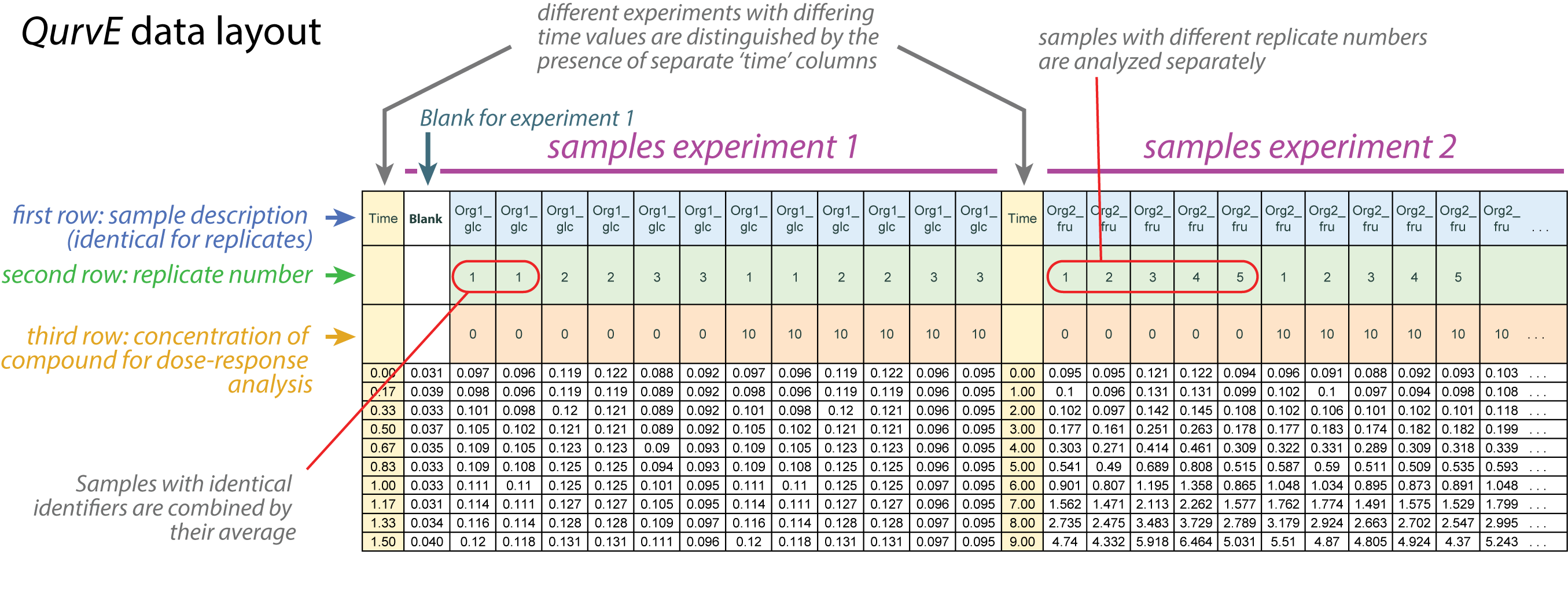To read data in custom format, run:

grodata <- read_data(data.growth = 'path_to_data_file',
csvsep = ';', # or ','
dec = '.', # or ','
sheet.growth = 1, # number (or "name") of the EXCEL file sheet containing data
subtract.blank = TRUE,
calib.growth = NULL)

The data.growth argument takes the path to the file or the name of an R dataframe object containing experimental data in custom format. csvsep specifies the separator symbol (only required for .csv files; default: ';'). dec is the decimal separator (only required for .csv, .tsv, or .txt files; default: '.'). If an Excel file format is used, sheet.growth specifies the number or name (in quotes) of the sheet containing the data.
If subtract.blank = TRUE, columns with name ‘blank’ will be combined by their row-wise average, and the mean values will be subtracted from the measurements of all remaining samples. For the calib.growth argument, a formula can be provided in the form ‘y = function(x)’ (e.g., calib.growth = 'y = x * 2 + 0.5') to transform growth measurement values.

## Tidy format

The QurvE package is designed to be flexible and user-friendly, and it is fully compatible with data in ‘tidy’ format. This format, also known as ‘long’ format, is a standard way of organizing data that suits many types of analyses and visualizations in R.

In tidy format, each row is an observation and each column is a variable. In the context of QurvE, your data should include the following columns:

• “Time”: This column should contain the time values for your observations.

• “Description”: This column should contain a description of the sample. This could be a combination of organism and condition or any other relevant descriptor.

• “Values”: This column should contain the measurement values for your experiment (e.g., optical density, cell count, etc.).

• “Replicate” (optional): If you have multiple replicate measurements for the same condition (“Description” labels), you can indicate the replicate number in this column.

• “Concentration” (optional): If there’s a compound of interest added to the sample, you can record its concentration in this column.

To load your tidy format data into QurvE, use the read_data function in the following way:

grodata <- read_data(data.growth = 'path_to_tidy_data_file',
csvsep = ';', # or ','
dec = '.', # or ','
sheet.growth = 1, # number (or "name") of the EXCEL file sheet containing data
subtract.blank = TRUE,
calib.growth = NULL)

This code block works the same way as for the custom format described above; QurvE detects automatically if data is in tidy format. Be sure to replace 'path_to_tidy_data_file' with the path to your own data file.

Note: Always ensure your data meets the tidy data standard. This means that every variable has its own column, every observation has its own row, and every value has its own cell. Additionally, make sure that your column headers exactly match those specified above, as QurvE will look for these specific headers when processing your data.

## Data parser

The data generated by culture devices (e.g., plate readers) from different manufacturers come in different formats. If these data are to be used directly, they must first be “parsed” from the plate reader into the QurvE standard format. In this scenario, sample information must be provided in a separate table that maps samples with their respective identifiers.The mapping table must have the following layout (Figure @ref(fig:mapping-layout)):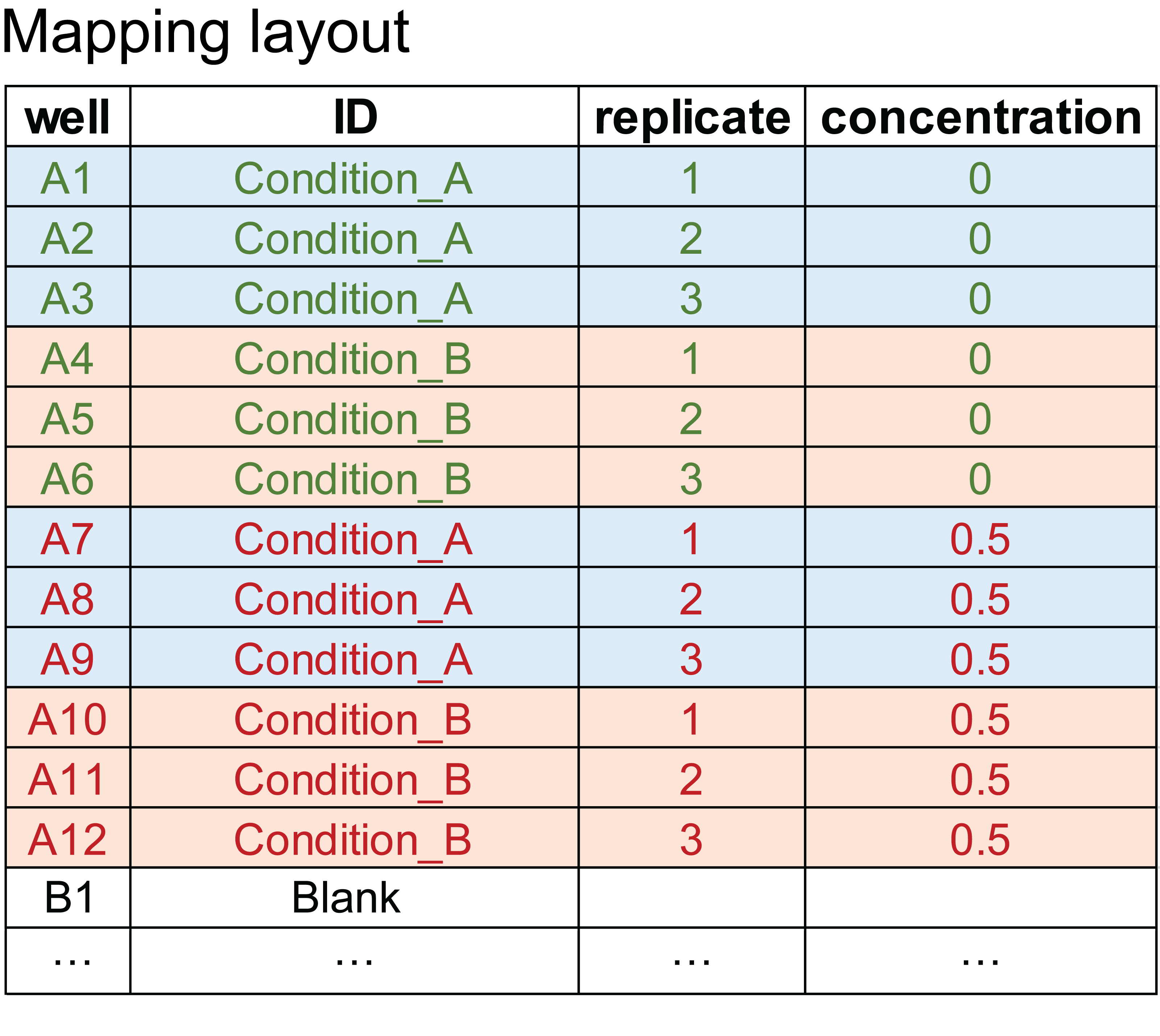Data parser mapping layout

To parse data, run:

grodata <- parse_data(data.file = 'path_to_data_file',
map.file = 'path_to_mapping_file',
software = 'used_software_or_device',
csvsep.data = ';', # or ','
dec.data = '.', # or ','
csvsep.map = ';', # or ','
dec.map = '.', # or ','
sheet.data = 1, # number (or "name") of the EXCEL file sheet containing data
sheet.map = 1, # number (or "name") of the EXCEL file sheet containing
# mapping information
subtract.blank = TRUE,
calib.growth = NULL,
convert.time = NULL)

The data.file argument takes the path to the file containing experimental data exported from a culture device, map.file the path to the file with mapping information. With software, you can specify the device (or software) that was used to generate the data. csvsep.data and csvsep.map specify the separator symbol for data and mapping file, respectively (only required for .csv files; default: ';'). dec.data and dec.map are the decimal separator used in data and mapping file, respectively (only required for .csv, .tsv, or .txt files; default: '.'). If an Excel file format is used for both or one of data or mapping file, sheet.data and/or sheet.map specify the number or name (in quotes) of the sheet containing the data or mapping information, respectively. If the same Excel file contains both data and mapping information in different worksheets, the file path needs to be specified for both data.fileand map.file. If subtract.blank = TRUE, samples with name ‘blank’ will be combined by their row-wise average, and the mean values will be subtracted from the measurements of all remaining samples. The argument convert.time accepts a function ‘y = function(x)’ to transform time values (e.g., convert.time = 'y = x/3600' to convert seconds to hours).

If more than one read type is identified in the provided data file, the user will be prompted to specify which measurements belong to growth, fluorescence, and fluorescence2, respectively.

# Run a complete growth analysis workflow

QurvE reduces all computational steps required to create a complete growth profiling to two steps, read data and run workflow.

library(QurvE)

we load experimental data from the publication Wirth & Nikel (2021) in which Pseudomonas putida KT2440 and an engineered strain were tested for their sensitivity towards the product 2-fluoromuconic acid:

grodata <- read_data(data.growth = system.file("2-FMA_toxicity.csv",
package = "QurvE"), csvsep = ";")

The created object grodata can be inspected with View(grodata). It is a list of class grodata containing:

• a time matrix with 66 rows, each corresponding to one sample in the dataset, and 161 columns, i.e., time values for each sample.

• a growth data frame with 66 rows and 161+3 columns. The three additional columns contain the sample identifiers condition, replicate, and concentration.

• fluorescence1 (here: NA)

• fluorescence2 (here: NA)

• norm.fluorescence1 (here: NA)

• norm.fluorescence2 (here: NA)

• expdesign, a data frame containing the label, condition, replicate, and concentration for each sample:

head(grodata$expdesign) #> label condition replicate concentration #> 1 KT2440 | 1 | 90 KT2440 1 90 #> 2 KT2440 | 1 | 70 KT2440 1 70 #> 3 KT2440 | 1 | 50 KT2440 1 50 #> 4 KT2440 | 1 | 25 KT2440 1 25 #> 5 KT2440 | 1 | 20 KT2440 1 20 #> 6 KT2440 | 1 | 15 KT2440 1 15 We can plot the raw data. Applying the generic plot() function to grodata objects calls the function plot.grodata().: plot(grodata, data.type = "growth", log.y = FALSE, x.lim = c(NA, 32), legend.position = "right", exclude.conc = c(50, 70, 90), basesize = 10, legend.ncol = 1, lwd = 0.7)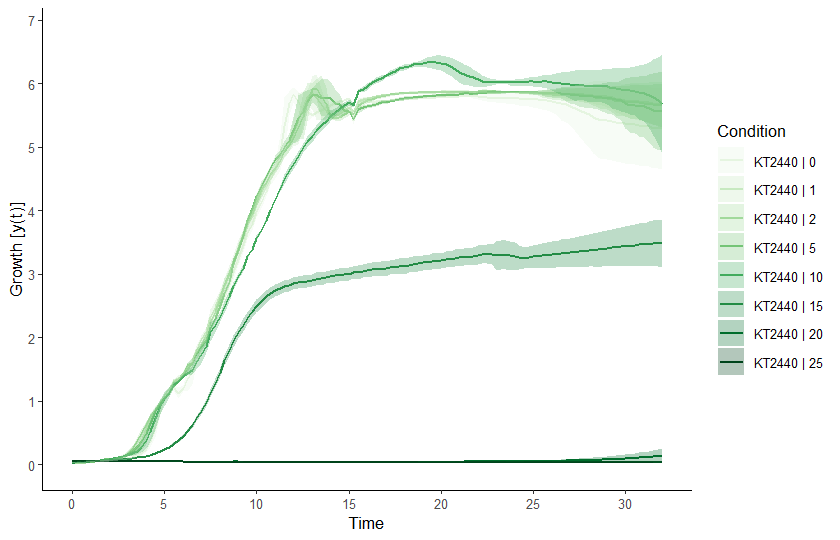Raw data plot. Conditions can be selected or deselected using the names = c('grp1', 'grp2') argument or exclude.nm = c('grp3', 'grp4') argument, respectively. Similarly, concentrations can be (de-selected) via the conc and exclude.conc arguments. To plot individual samples instead of grouping replicates, add mean = FALSE. See ?plot.grodata for further options. ## Run Workflow To perform a complete growth profiling of all samples in the input dataset, we call the growth.workflow() function on the grodata object. With supress.messages = TRUE, we avoid printing information about every sample’s fit in the sample to the console. By default, the selected response parameter to perform a dose-response analysis is ‘mu.linfit’. To choose a different parameter, provide the argument dr.parameter = 'choice'. A list of appropriate parameters is provided within the function documentation (?growth.workflow). grofit <- growth.workflow(grodata = grodata, fit.opt = "a", ec50 = TRUE, suppress.messages = TRUE, export.res = FALSE, # Prevent creating TXT table and RData files with results parallelize = FALSE) # Use only one available CPU core If option export.res is set to TRUE, tab-delimited .txt files summarizing the computation results are created, as well as the grofit object (an object of class grofit) as .RData file. This object (or the .RData file) contains all raw data, fitting options, and computational results. Figure @ref(fig:grofit-container) shows the structure of the generated grofit object. In RStudio, View(grofit) allows interactive inspection of the data container. If you want to create a report summarizing all computational results including a graphical representation of every fit, provide the desired output format(s) as report = 'pdf', report = 'html', or report = c('pdf', 'html'). The advantage of having the report in HTML format is that every figure can be exported as (editable) PDF file. In the spirit of good scientific practice (data transparency), I would encourage anyone using QurvE to attach the .RData file and generated reports to their publication. Arguments that are commonly modified:  fit.opt Which growth fitting methods to perform; a string containing 'l' for linear fits, 's' for spline fits, 'm' for model fits, or 'a'(the default) for all three methods. Combinations can be also given as a vector of strings, e.g., c('l', 's'). model.type Which growth models to apply; a string containing one of, or a vector of strings containing any combination of ‘logistic’, ‘richards’, ‘gompertz’, ‘gompertz.exp’, ‘huang’, and ‘baranyi’. log.y.lin log.y.spline log.y.model Should Ln(y/y0) be applied to the growth data for the respective fits? biphasic Extract growth parameters for two different growth phases (as observed with, e.g., diauxic shifts) interactive Controls interactive mode. If TRUE, each fit is visualized in the Plots pane and the user can adjust fitting parameters and confirm the reliability of each fit per sample nboot.gc Number of bootstrap samples used for nonparametric growth curve fitting. See ?growth.gcBootSpline for details. dr.method Define the method used to perform a dose-responde analysis: smooth spline fit ('spline') or model fitting ('model', the default). See section 4 dr.parameter The response parameter in the output table to be used for creating a dose response curve. See ?growth.drFit for further details. Please consult ?growth.workflow for further arguments to customize the workflow.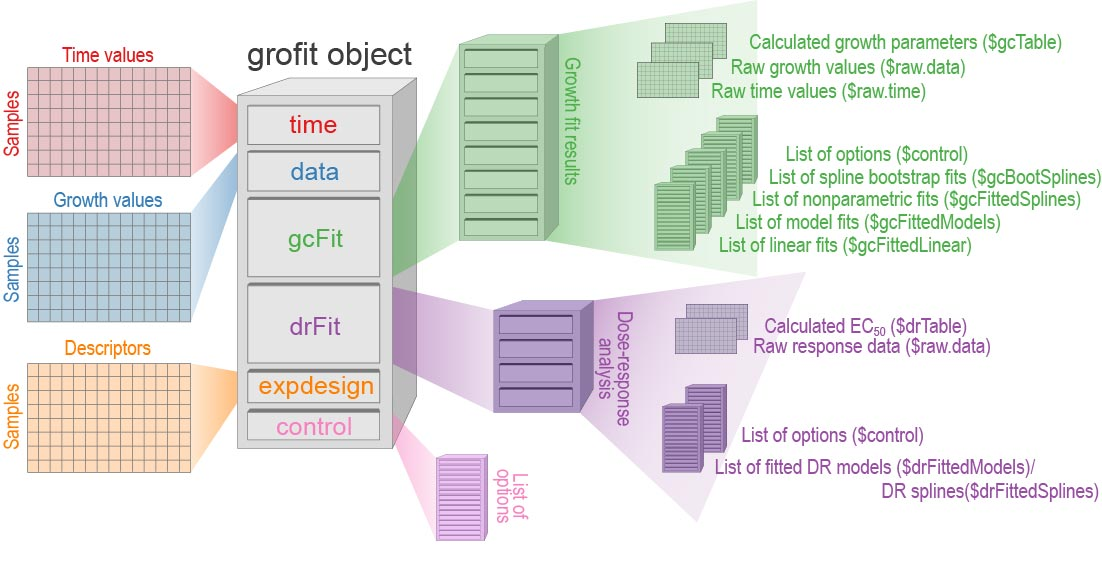Internal structure of a grofitobject generated by growth.workflow(). ## Tabular results A grofit object contains two tables summarizing the computational results: - grofit$gcFit$gcTable lists all calculated physiological parameters for every sample and fit - grofit$drFit$drTable contains the results of the dose-response analysis # show the first three rows and first 14 columns of gcTable gcTable <- grofit$gcFit$gcTable gcTable[1:3, 1:14] TestId AddId concentration reliability_tag used.model log.x log.y.lin log.y.spline 1 KT2440 1 90 TRUE FALSE TRUE TRUE 2 KT2440 1 70 TRUE FALSE TRUE TRUE 3 KT2440 1 50 TRUE FALSE TRUE TRUE log.y.model nboot.gc mu.linfit tD.linfit lambda.linfit dY.linfit 1 TRUE 0 0 0 2 TRUE 0 0 0 3 TRUE 0 0 0 # Show drTable. The function as.data.frame() ensures that it is shown in table format. drTable <- as.data.frame(grofit$drFit$drTable) Additionally, the dedicated functions table_group_growth_linear(), table_group_growth_model(), and table_group_growth_spline() allow the generation of grouped results tables for each of the three fit types with averages and standard deviations. The column headers in the resulting data frames are formatted with HTML for visualization in shiny and with DT::datatable(). A summary of results for each individual fit can be obtained by applying the generic function summary() to any fit object within grofit. ## Visualize results Several generic plot() methods have been written to allow easy plotting of results by merely accessing list items within the grofit object structure (Figure @ref(fig:grofit-container)). ### Grouped spline fits Applying plot() to the grofit object produces a figure of all spline fits performed as well as the first derivative (slope) over time. The generic function calls plot.grofit() with data.type = 'spline' and thus, the same options are available as described for Figure @ref(fig:raw-data-plot). plot(grofit, data.type = "spline", log.y = TRUE, deriv = TRUE, conc = c(0,5,10,15,20), legend.position = "right", legend.ncol = 1, x.lim = c(NA, 32), y.lim = c(0.01,NA), n.ybreaks = 10, basesize=10, lwd = 0.7)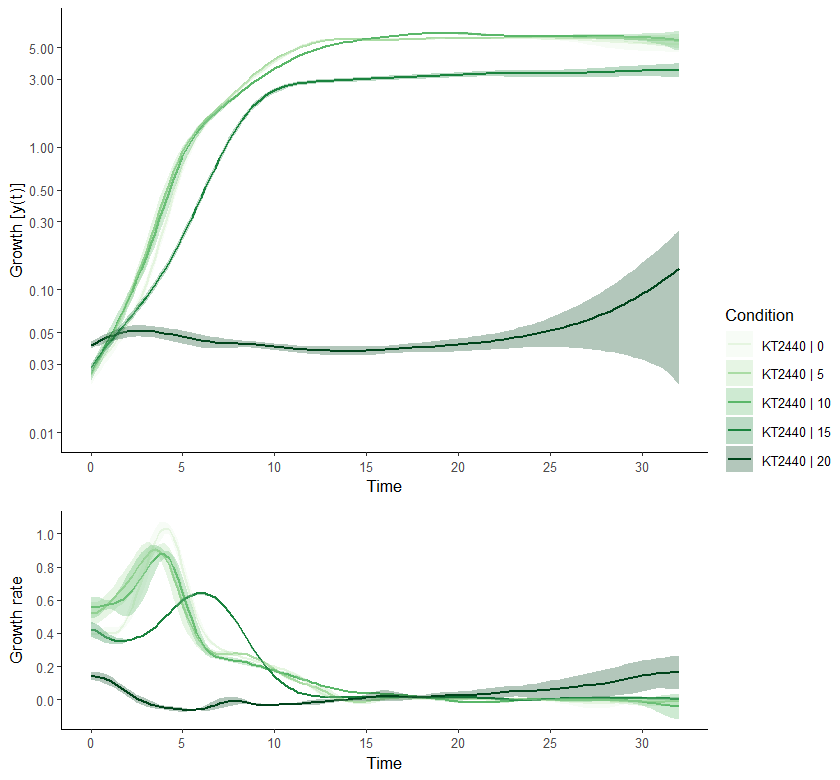Combined plot of all spline fits performed. In addition to the options available with data.type = 'raw', further arguments can be defined that control the appearance of the secondary panel showing the slope over time. See ?plot.grofit for all options. ### Compare growth parameters A convenient way to compare the performance of different organisms under different conditions is to plot the calculated growth parameters by means of the function plot.parameter(). # Parameters obtained from linear regression plot.parameter(grofit, param = "mu.linfit", basesize = 10, legend.position = "bottom") plot.parameter(grofit, param = "dY.linfit", basesize = 10, legend.position = "bottom") # Parameters obtained from nonparametric fits plot.parameter(grofit, param = "mu.spline", basesize = 10, legend.position = "bottom") plot.parameter(grofit, param = "dY.spline", basesize = 10, legend.position = "bottom") # Parameters obtained from model fits plot.parameter(grofit, param = "mu.model", basesize = 10, legend.position = "bottom") plot.parameter(grofit, param = "dY.orig.model", basesize = 10, legend.position = "bottom")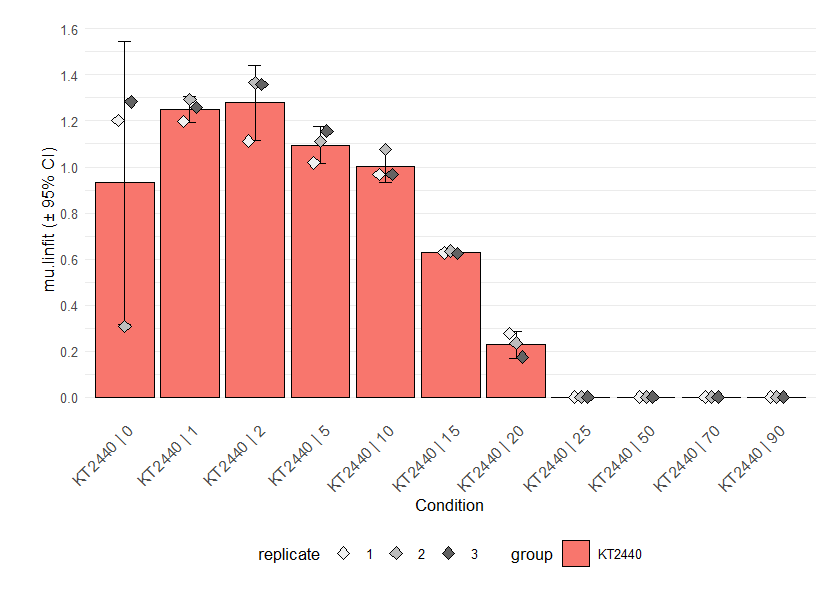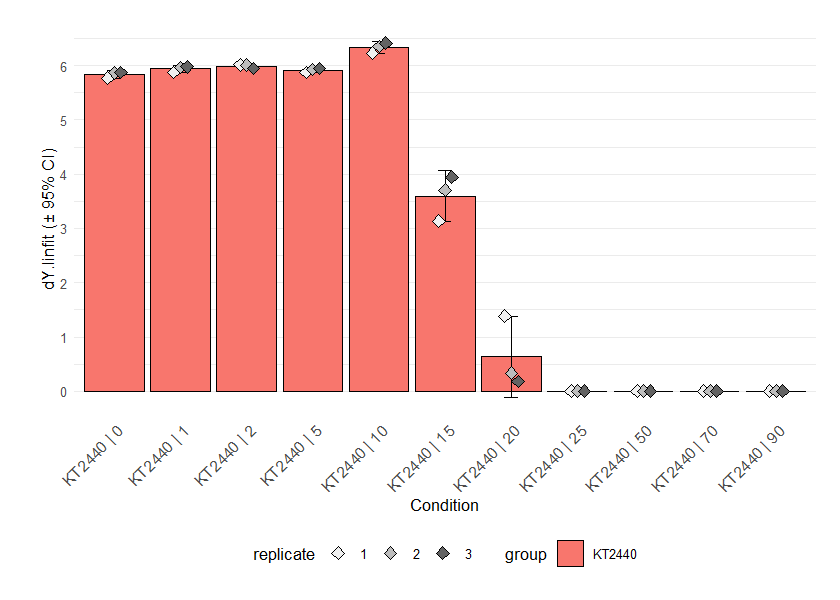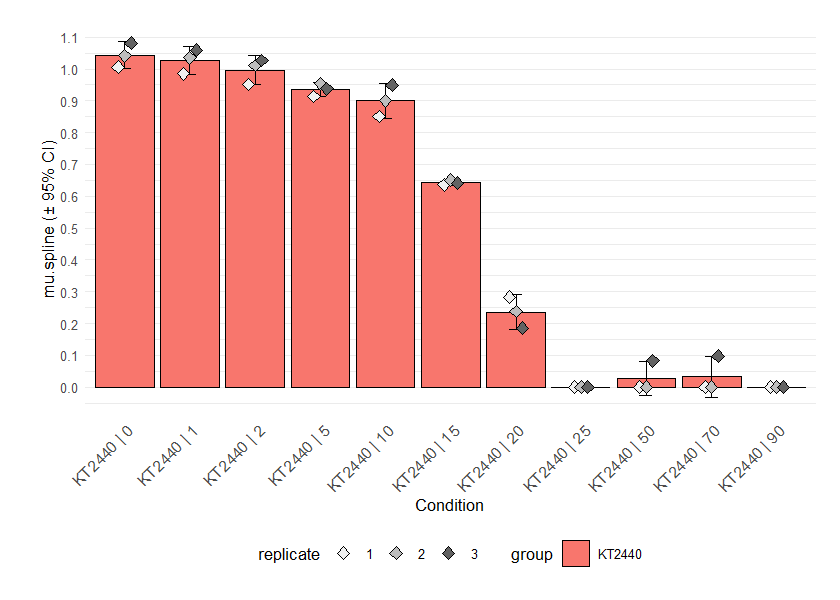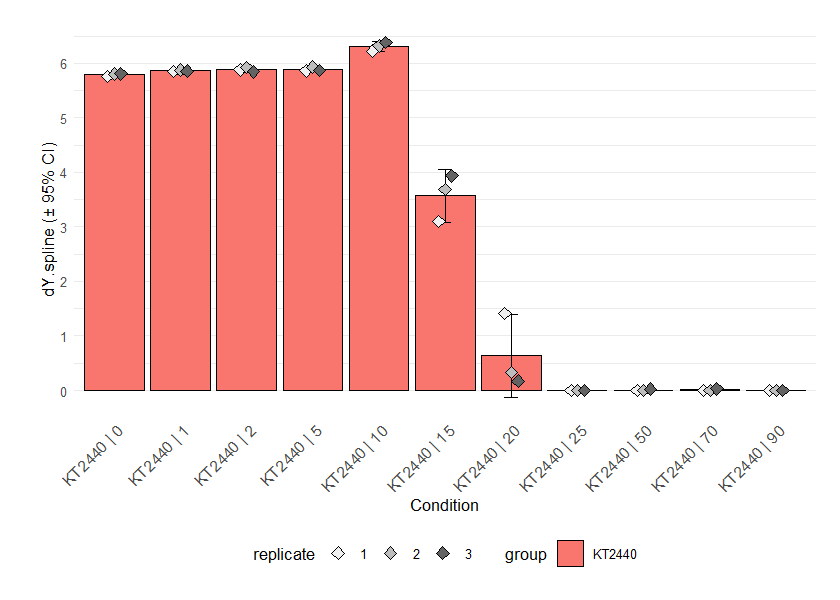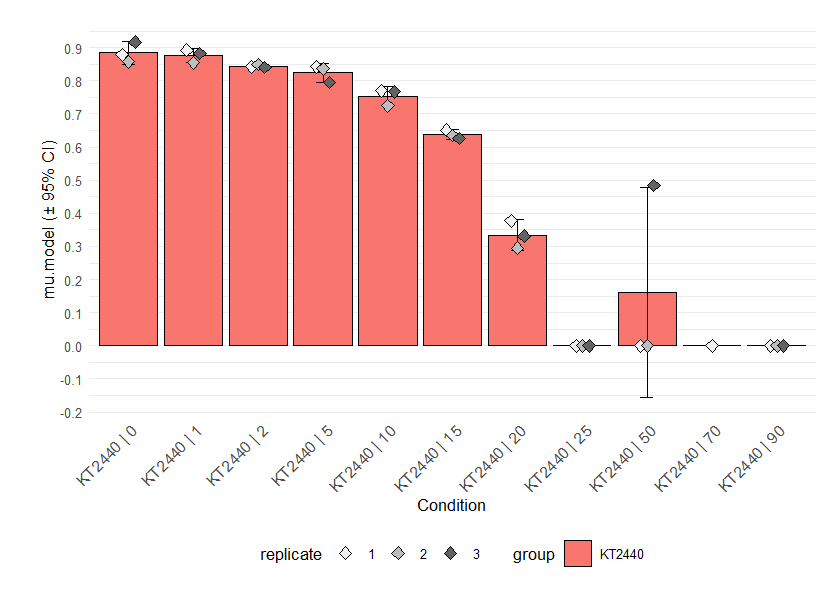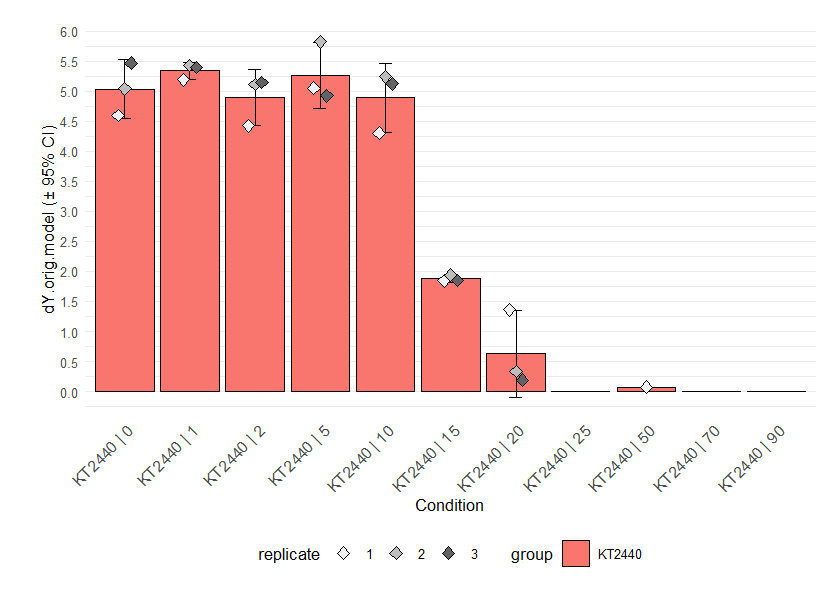Parameter plots. If mean = TRUE, the results of replicates are combined and shown as their mean ± 95% confidence interval. As with the functions for combining different growth curves, the arguments name, exclude.nm, conc and exclude.conc allow (de)selection of specific samples or conditions. Since we applied growth models to log-transformed data, calling ‘dY.orig.model’ or ‘A.orig.model’ instead of ‘dY.model’ or ‘A.model’ provides the respective values on the original scale. For linear and spline fits, this is done automatically. For details about this function, run ?plot.parameter. From the parameter plot for ´mu.linfit´ (the growth rates determined with linear regression), we can see that there is an outlier for strain KT2440 at concentration 0. We can plot the individual fits for this condition to find out if this is due to the fit quality: plot(grofit$gcFit$gcFittedLinear$KT2440 | 1 | 0, cex.lab = 1.2,
cex.axis = 1.2)
plot(grofit$gcFit$gcFittedLinear\$KT2440 | 2 | 0, cex.lab = 1.2,
cex.axis = 1.2)